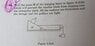# A classic string and pulley problem

sahilmm15
I tried hard but was not able to make progress. Problem no 33.

#### Attachments

•IMG_20201224_220234.jpg
43.9 KB · Views: 64

Homework Helper
Gold Member
Trying hard means that you have some attempts to show. Please show them or at least share your thoughts about how far you got and where you got stuck.

•etotheipi
sahilmm15
I have drawn all the Free body diagrams finding difficulty in equating them
The diagonal arrows are forces from normal reaction. I didn't wrote it. Sorry for any inconveniences.

Homework Helper
Gold Member
Drawing free body diagrams (FBDs) doesn't get you very far unless you write equations based on Newton's second law appropriate to them. You have correctly identified that you have three systems, the hanging mass, the wedge and the block. What equations can you get out out of them? Hint: ##\vec F_{\text{net}}=m\vec a.## Write expressions for each of the left and right sides for each of the 3 systems.

•Lnewqban
Homework Helper
Gold Member
Imagine the triangular box as being anchored to ground and the smaller block freely slipping over it.
How much acceleration the smaller block would reach?
What forces would induce that acceleration and how strong they would be?

•1)You're free body force diagram (FBD) for falling mass M looks good.

2)The other two don't look right. For example, the triangular mass M' is accelerating with same acceleration as M to the right. There is also a reaction force on M' due to mass m to consider.

3)To solve this problem, you need to think about what it means acceleration-wise for the m not to slipping over M'

4)Note, in these kind of questions, although you'll always be applying 'F=ma", there will often be more than one way of getting the right answer!

•Lnewqban
Homework Helper
Gold Member
2022 Award
The diagonal arrows are forces from normal reaction. I didn't wrote it. Sorry for any inconveniences.
Without some stated notation, I'm not quite sure what your diagrams mean. You show some actual forces, like mg, but also resultants, like ma, and omit to label the normal forces. It would be clearer had you got as far as writing equations.

Please label all actual forces with variable names and either remove resultant forces or distinguish them by using a double arrowhead.
Or just post the equations you get for force balance,

Mentor
If the mass m is not slipping relative to the wedge M', then it must be moving with the exact same acceleration as M'.

sahilmm15
Drawing free body diagrams (FBDs) doesn't get you very far unless you write equations based on Newton's second law appropriate to them. You have correctly identified that you have three systems, the hanging mass, the wedge and the block. What equations can you get out out of them? Hint: ##\vec F_{\text{net}}=m\vec a.## Write expressions for each of the left and right sides for each of the 3 systems.
Well , after some time (30 minutes) I was able to solve the problem. I completely left the problem for 1 hour so that my brain can work on it subconsciously(as suggested by one of my mentors in other thread). It came to my surprise that I solved it easily. Shall I provide you my work?

Last edited:
•sahilmm15
1)You're free body force diagram (FBD) for falling mass M looks good.

2)The other two don't look right. For example, the triangular mass M' is accelerating with same acceleration as M to the right. There is also a reaction force on M' due to mass m to consider.

3)To solve this problem, you need to think about what it means acceleration-wise for the m not to slipping over M'

4)Note, in these kind of questions, although you'll always be applying 'F=ma", there will often be more than one way of getting the right answer!

#### Attachments

•sahilmm15
In step 2 of my answer it states "to keep 'm' at rest".And the statement I wrote in step 1 was for step 2. Sorry for any inconveniences.

sahilmm15
In step 2 of my answer it states "to keep 'm' at rest".And the statement I wrote in step 1 was for step 2. Sorry for any inconveniences.
Also for triangular block I didn't draw the fbd but directly wrote the equation.

Homework Helper
Gold Member
2022 Award
##M=M\tan(\theta)+(M'+m)\tan(\theta)##
##M=\frac{M'+m}{\cot(\theta)}##
The final step is wrong.

sahilmm15
The final step is wrong.
Thanks for your reply, I appreciate it. It should be cot(x) - ##1## in the denominator. It was an calculation error.